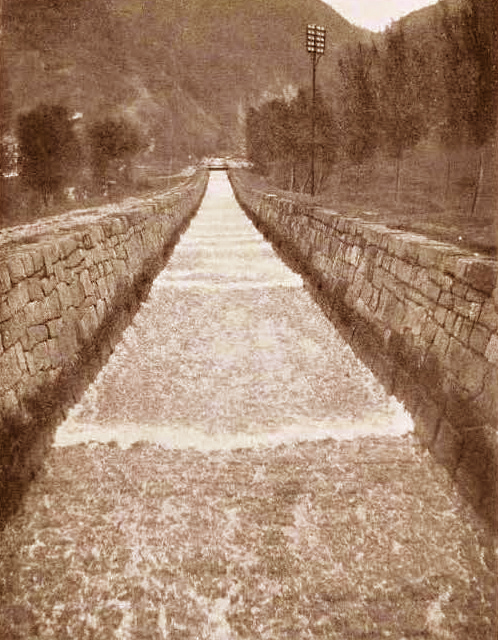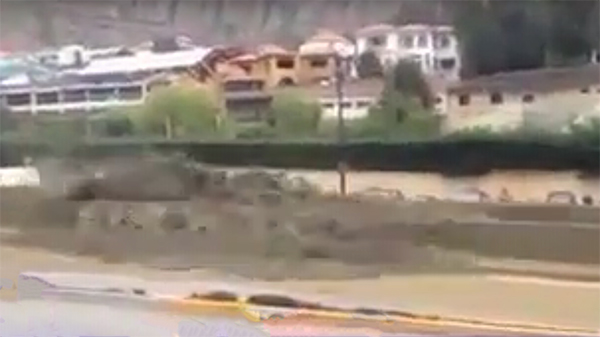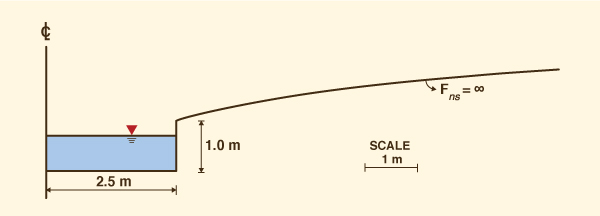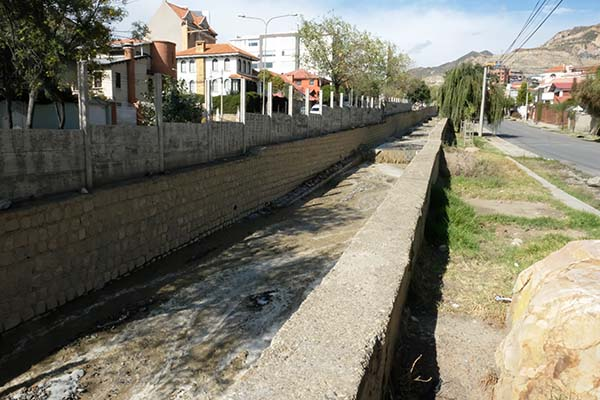DESIGN OF A STABLE CHANNEL ON A STEEP SLOPE

USING THE EXPONENT OF THE RATING CURVE

Victor M. Ponce 1 and Vassiliki Boulomytis 2

1 San Diego State University, San Diego, California, USA

2 Instituto Federal de São Paulo, Campus Caraguatuba, São Paulo, Brazil



 ABSTRACT.  The design of a stable channel to be hydraulically stable is governed by the well-known Vedernikov criterion. However, it can be shown that channel stability depends on the shape of the cross section, whether it is trapezoidal, rectangular, or triangular. For a given section, there is a unique relationship between the exponent β of the rating curve Q - A (flow rate vs flow area) and the value of V/F, in which V = Vedernikov number, and F = Froude number. In this article we use the online calculator15b, which calculates the value of β and the corresponding Vedernikov number for a rectangular, trapezoidal, or triangular cross section. Three series of tests are carried out for a hypothetical steep channel, keeping constant the flow rate Q, Manning friction coefficient n, and the bottom slope S, while varying the value of the side slope z: (a) 0.25; (b) 0.5, and (c) 1.0. It is concluded that by judiciously reducing the bottom width b, the Vedernikov number V decreases more quickly to values less than 1 for the smallest values of the side slope z in the range 0.25 ≤ z < 1.

1.  INTRODUCTION

The design of a lined canal on a steep slope to be hydraulically stable is governed by the well-known Vedernikov criterion (Ponce, 2014). However, it can be shown that this depends on the shape of the cross section, whether it is trapezoidal, rectangular, or triangular. For a given section, there is a unique relationship between the exponent β of the rating curve (the discharge Q vs flow area A relation) and the value of V/F, in which V = Vedernikov number, and F = Froude number.

Herein, we use the online calculator calculator15b, which calculates the value of β for a trapezoidal, rectangular, or triangular cross section. Unstable flow theory indicates that stability is achieved for values of β greater than but close to 1. At the lower limit, for β = 1, the section is inherently stable, that is, stable for any value of the Froude number (Ponce and Porras, 1995). We calculate the value of β for a series of sections, keeping constant the flow rate Q, friction coefficient n, and bottom slope S, and varying the side slope z (z H: 1 V) and, therefore, the flow depth y. The calculator determines the Froude F and Vedernikov V numbers, and the associated value of β. The optimal design section is that which corresponds to the lowest value of β compatible with the planned cost of the project. This cost is a function of the depth of excavation that is required to ensure that the flow remains stable, that is, to assure that V < 1.

2.  THEORY

The theory of hydrodynamic stability of open-channel flow is due to Vedernikov, who introduced the concept of the number bearing his name (Vedernikov, 1945; Powell, 1948). According to this theory, the Vedernikov number is the relationship between the relative speed of the kinematic wave and the relative speed of the dynamic wave (Ponce, 1991). For V < 1, the flow is stable; for V > 1 the flow is unstable. This latter condition is frequently associated with the occurrence of roll waves (Fig. 1).Cornish
 Fig. 1  Roll waves observed in a canal in the Swiss Alpsat the turn of the 20th century (Cornish, 1907).

In certain cases, the roll waves may be of such magnitude to threaten life and property, as shown by the recent experience in channeled rivers built in La Paz, Bolivia in the past two to three decades (Fig. 2) (Ponce and Choque Guzman, 2019). Therefore, it is imperative to design canals to avoid or reduce the incidence of roll waves. As shown here, this may be achieved by designing the shape of the cross section to reduce the value of β so that the Vedernikov number remains less than the threshold value V = 1.

[Click on top of the figure to watch the video]Jorge Molina
 Fig. 2  Occurrence of roll waves in the channelized section of the Huayñajahuira river,La Paz, Bolivia, on February 24, 2016.

3.  RELATIONSHIP BETWEEN β AND V/F

Ponce (2014) has determined the relation between the exponent β of the rating curve (Q = α Aβ ) and the value of V/F:

 V β   =   1  +   ______                       F
(1)

For V = 1, the neutrally stable Froude number Fns is:

 1 Fns   =     ______                  β - 1
(2)

Table 1 shows corresponding values of β and Fns for three types of cross-sectional shape and two types of friction. Figure 3 shows the shape of the inherently stable channel (Ponce and Porras, 1995).

 Table 1.  Values of β and Fns corresponding tothree typical cross-sectional shapes. Cross-sectional shape Friction β Fns Hydraulically wide Manning 5/3 3/2 Chezy 3/2 2 Triangular Manning 4/3 3 Chezy 5/4 4 Inherently stable Manning or Chezy 1 ∞Ponce and Porras
 Fig. 3  Shape of an inherently stable channel.

4.  ONLINE CALCULATOR

The online calculator onlinechannel15b calculates the value of β, the exponent of the rating curve, corresponding to a rectangular, trapezoidal, or triangular cross-sectional shape. Input data is the following:

1. Bottom width b

2. Flow depth y

3. Side slope z1

4. Side slope z2

5. Manning's n

6. Bottom slope S

Output results are the following:

1. Wetted perimeter P

2. Top width T

3. Flow area A

4. Hydraulic radius R   [R = A / P ]

5. Hydraulic depth D   [D = A / T ]

6. Discharge Q

7. Flow velocity v   [v = Q / A ]

8. Froude number F   [F = v /(gD1/2)]

9. Rating exponent β   [Eq. 1]

10. Neutrally stable Froude number Fns   [Eq. 2]

11. Vedernikov number V   [V = (β - 1) F ]

The results are obtained by the trial and error described here. Given the selected (constant) input values of z1, z2, n and S, the chosen design discharge Q is obtained by trial and error using the calculator. The procedure consists of varying the bottom width b of the trapezoidal channel, within an appropriate range, to determine the value of flow depth y that exactly matches the chosen design discharge Q. Output results consist of v, F, β, Fns and V. The results are analyzed, including project cost considerations, to estimate the design bottom width b that best satisfies the Vedernikov stability criterion: V ≤ 1.

5.  THE UNSTABLE CHANNEL

A hypothetical example of an unstable channel is presented herein. Assume a channel of rectangular cross-section, of width b = 6 m. The design discharge is Q = 100 m3/s, the Manning coefficient is n = 0.025 (masonry-lined channel), and the bottom slope is S = 0.06. These data approximately simulate the conditions in the Huay�ajahuira and Achumani channelized rivers of (the city of) La Paz, Bolivia (Figs. 4 and 5). In these channels, the occurrence of roll wave events has been documented with some regularity in the past several years (Ponce and Choque Guzman, 2019).Fig. 4  The channelized Huayñajahuira river, La Paz, Bolivia.Fig. 5  The channelized Achumani river, La Paz, Bolivia.

Figure 6 shows the output of onlinechannel15b. For the given flow conditions, the calculated Vedernikov number is: V = 1.48; that is, the flow is unstable.Fig. 6  Calculations for the case of unstable flow in a steep channel.

6.  TESTING PROGRAM

The objective of the testing program is to determine the hydraulic conditions in a series of alternative channel sections for which the Vedernikov number covers the range V ≷ 1. This is obtained by specifying a trapezoidal cross-section (z > 0) and varying the bottom width b within a suitable range.

The testing program considers the following three series of trapezoidal cross-sections:

• z = 0.25;

• z = 0.5; and

• z = 1.0.

The results of the calculation are shown in Tables 2 to 4. It is concluded that when reducing the bottom width b in the range 5 ≥ b ≥ 1, the Vedernikov number V decreases more quickly to values less than 1 for the smallest values of the side slope z in the range 0.25 ≤ z ≤ 1. We note that the lowest value of V  (V = 0.55) is obtained for the case of z = 0.25 and b = 1 (Table 2).

 Table 2. Results for Series A  (z = 0.25). Q = 100 m3/s n = 0.025 S = 0.06 Variable Bottom width b (m) 5 4 3 2 1 Input y 1.754 2.078 2.581 3.408 4.769 Output flow variables P 8.615 8.283 8.320 9.806 10.83 T 5.877 5.039 4.290 3.704 3.384 A 9.539 9.391 9.408 9.719 10.45 R 1.107 1.133 1.130 1.076 0.965 D 1.623 1.863 2.192 2.624 3.089 Results v 10.48 10.65 10.63 10.29 9.569 F 2.62 2.49 2.29 2.02 1.73 β 1.56 1.53 1.48 1.40 1.32 Fns 1.76 1.87 2.07 2.45 3.12 V * 1.48 1.32 1.10 0.82 0.55 * Two stable values of V  were found, shown in bold.

 Table 3. Results for Series B  (z = 0.5). Q = 100 m3/s n = 0.025 S = 0.06 Variable Bottom width b (m) 5 4 3 2 1 Input y 1.643 1.894 2.249 2.763 3.503 Output flow variables P 8.673 8.235 8.028 8.178 8.832 T 6.643 5.894 5.249 4.763 4.503 A 9.564 9.369 9.276 9.343 9.639 R 1.102 1.137 1.155 1.142 1.091 D 1.439 1.589 1.767 1.961 2.140 Results v 10.45 10.67 10.78 10.70 10.38 F 2.78 2.70 2.59 2.44 2.26 β 1.56 1.53 1.49 1.43 1.35 Fns 1.76 1.86 2.02 2.30 2.77 V * 1.57 1.44 1.27 1.05 0.81 * One stable value of V  was found, shown in bold.

 Table 4. Results for Series C:  (z = 1). Q = 100 m3/s n = 0.025 S = 0.06 Variable Bottom width b (m) 5 4 3 2 1 Input y 1.509 1.689 1.922 2.224 2.613 Output flow variables P 9.268 8.777 8.436 8.290 8.390 T 8.018 6.844 6.844 6.448 6.226 A 9.822 9.608 9.460 9.394 9.440 R 1.059 1.094 1.121 1.133 1.125 D 1.225 1.302 1.382 1.456 1.516 Results v 10.18 10.40 10.57 10.64 10.59 F 2.93 2.91 2.87 2.81 2.74 β 1.55 1.52 1.49 1.44 1.38 Fns 1.79 1.89 2.03 2.25 2.61 V * 1.63 1.54 1.41 1.24 1.05 * No stable values of V  were found.

It is noted that the Froude number F is a function of hydraulic depth D, and not of flow depth y (Section 4).

The examination of Tables 2 to 4 enables the following conclusions:

1. The smaller the value of z, the larger the value of D and, therefore, the smaller the value of F.

2. The values of β decrease sharply as the bottom width b decreases from 5 to 1, and slightly as the side slope z decreases from 1 to 0.25.

3. Within the tested range. the lower the value of z, the faster the Vedernikov number falls below 1.

4. The most stable section, that is, that with the smallest Vedernikov number below 1, corresponds to the smallest value of b and the smallest value of z, within the tested range.

For a given hydraulic condition, the lower Vedernikov V numbers correspond to the higher neutrally stable Froude numbers Fns  and, consequently, to larger hydraulic depths D. The higher the value of D, the lower the value of β (in the range β greater but close to 1), which favors hydrodynamic stability. In the limit, β = 1 constitutes an inherently stable cross section (Fig. 3), that is, stable for any value of Froude number F.

7.  CONCLUSIONS

The results of this study show that as the bottom width b decreases within the tested range 5 ≥ b ≥ 1, with side slope z > 0, the values of β and V decrease accordingly. The reduction in the Vedernikov number depends on the value of the side slope z, the reduction being faster (with the decrease in bottom width b) when z is smaller, within the tested range. In other words, the smaller the side slope z (within the tested range 0.25 ≤ z ≤ 1), the faster the Vedernikov number falls below 1, enhancing hydrodynamic stability. Therefore, it is concluded that the value of b that reduces the Vedernikov number below 1 depends on the value of z. The smallest b coupled with the smallest z result in the lowest V.

The online calculator onlinechannel15b is a useful tool for the analysis and design of lined canals and channels, with the objective of promoting hydrodynamic stability and, therefore, avoiding roll waves.

 Clickto watch a video with the content shown in this article.

REFERENCES

Cornish, V. 1907. Progressive waves in rivers. Journal of the Royal Geographical Society, Vol. 29, No. 1, January, 23-31.

Liggett, J. A. 1975. Stability. Chapter 6 in Unsteady Flow in Open Channels, K. Mahmood and V. Yevjevich, eds., Water Resources Publications, Ft. Collins, Colorado.

Ponce, V. M. 1991. New perspective on the Vedernikov number. Water Resources Research, Vol. 27, No. 7, 1777-1779, July.

Ponce, V. M., and P. J. Porras. 1995. Effect of cross-sectional shape on free-surface instability. Journal of Hydraulic Engineering, ASCE, Vol. 121, No. 4, April, 376-380.

Ponce, V. M. 2014. Chow, Froude, and Vedernikov. Proceedings, American Society of Civil Engineers (ASCE) World Environment and Water Resources Congress, June 1-5, 2014, Portland, Oregon.

Ponce, V. M. and B. Choque Guzmán, 2019. El control de ondas pulsantes en ríos canalizados. http://ponce.sdsu.edu/el_control_de_ondas_pulsantes.html   (Cited May 7, 2021).

Powell, R. W. 1948. Vedernikov's criterion for ultra-rapid flow. Transactions, American Geophysical Union, Vol. 29, No. 6, 882-886.

Vedernikov, V. V. 1945. Conditions at the front of a translation wave disturbing a steady motion of a real fluid, Dokl. Akad. Nauk SSSR, 48(4), 239-242.

 220118 19:00